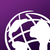# Take Minimum value of multiple raster using ArcPy

1212
7
07-12-2018 02:29 AMOccasional Contributor III

Hi all,

I have monthly raster for different parameters in three different folders. I am trying to take the minimum value of raster from each raster in two different parameters and solve the below equation. For that, I have written a code, but the code is producing an error.

It is giving an error in line no 35.

Sample data attached below

Code:

``````def main():
import arcpy
import os
arcpy.env.overwriteOutput = True
# Checkout extension
arcpy.CheckOutExtension("Spatial")

ws_in_APAR = r'E:\APAR'

ws_in_TSCALAR = r'E:\T_SCALAR'

ws_in_WSCALAR = r'E:\W_SCALAR'

ws_out_GPP = r'E:\GPP_1982'

# list "mean" rasters (r001_APAR so on)
arcpy.env.workspace = ws_in_APAR
lst_ras_APAR = arcpy.ListRasters()
print "lst_ras_APAR", lst_ras_APAR

# list "mean" rasters (r001_TSCALAR so on)
arcpy.env.workspace = ws_in_TSCALAR
lst_ras_TSCALAR = arcpy.ListRasters()
print "lst_ras_TSCALAR", lst_ras_TSCALAR

# list "mean" rasters (r001_WSCALAR so on)
arcpy.env.workspace = ws_in_WSCALAR
lst_ras_WSCALAR = arcpy.ListRasters()
print "lst_ras_WSCALAR", lst_ras_WSCALAR

for ras_name in lst_ras_APAR, lst_ras_TSCALAR, lst_ras_WSCALAR   :
ras_APAR = arcpy.Raster(os.path.join(ws_in_APAR, ras_APAR))
ras_TSCALAR = arcpy.Raster(os.path.join(ws_in_TSCALAR, ras_TSCALAR))
ras_WSCALAR = arcpy.Raster(os.path.join(ws_in_WSCALAR, ras_WSCALAR))

# calculate (ras_TSCALAR.minimum wil take the minimum value of perticular raster)
ras_GPP = (ras_APAR * 1.48) * (ras_TSCALAR.minimum * ras_WSCALAR.minimum)

# save raster
ras_num = ras_name[:3]
out_name_GPP = os.path.join(ws_out_GPP, 'r{0}_GPP'.format(ras_num))
ras_GPP.save(out_name_GPP)

if __name__ == '__main__':
main()‍‍‍‍‍‍‍‍‍‍‍‍‍‍‍‍‍‍‍‍‍‍‍‍‍‍‍‍‍‍‍‍‍‍‍‍‍‍‍‍‍‍‍‍‍‍‍‍‍‍‍‍‍‍‍‍‍‍‍‍‍‍‍‍‍‍‍‍‍‍‍‍‍‍‍‍‍‍‍‍‍‍‍‍‍‍‍‍‍‍‍‍‍‍‍‍‍‍‍‍‍‍‍‍‍‍‍‍‍‍‍‍‍‍‍‍‍‍‍‍‍‍‍‍‍‍‍‍‍‍‍‍‍‍‍‍‍‍‍‍‍‍‍‍‍‍‍‍‍‍‍‍‍‍‍‍‍‍‍``````

Error message:

``````Traceback (most recent call last):
File "E:\GPP.py", line 64, in <module>
main()
File "E:\GPP.py", line 47, in main
ras_APAR = arcpy.Raster(os.path.join(ws_in_APAR, ras_APAR))
UnboundLocalError: local variable 'ras_APAR' referenced before assignment‍‍‍‍‍‍‍‍‍‍‍‍‍‍‍‍‍‍``````
Tags (3)
1 Solution

Accepted SolutionsbyEsri Esteemed Contributor

Have a try with this code (change the paths on lines 8 to 11):

``````def main():
import arcpy
import os
arcpy.env.overwriteOutput = True
# Checkout extension
arcpy.CheckOutExtension("Spatial")

ws_in_APAR = r'C:\GeoNet\MinMultiRas\APAR'
ws_in_TSCALAR = r'C:\GeoNet\MinMultiRas\T_SCALAR'
ws_in_WSCALAR = r'C:\GeoNet\MinMultiRas\W_SCALAR'
ws_out_GPP = r'C:\GeoNet\MinMultiRas\GPP_1982'

# list "mean" rasters (r001_APAR so on)
arcpy.env.workspace = ws_in_APAR
lst_ras_APAR = arcpy.ListRasters()
print "lst_ras_APAR", lst_ras_APAR

# list "mean" rasters (r001_TSCALAR so on)
arcpy.env.workspace = ws_in_TSCALAR
lst_ras_TSCALAR = arcpy.ListRasters()
print "lst_ras_TSCALAR", lst_ras_TSCALAR

# list "mean" rasters (r001_WSCALAR so on)
arcpy.env.workspace = ws_in_WSCALAR
lst_ras_WSCALAR = arcpy.ListRasters()
print "lst_ras_WSCALAR", lst_ras_WSCALAR

if len(lst_ras_APAR) == len(lst_ras_TSCALAR) == len(lst_ras_WSCALAR):
print("each list has equal amount of rasters, let's assume they match...")

for i in range(len(lst_ras_APAR)):
ras_APAR = arcpy.Raster(os.path.join(ws_in_APAR, lst_ras_APAR[i]))
ras_TSCALAR = arcpy.Raster(os.path.join(ws_in_TSCALAR, lst_ras_TSCALAR[i]))
ras_WSCALAR = arcpy.Raster(os.path.join(ws_in_WSCALAR, lst_ras_WSCALAR[i]))

for ras_path in [os.path.join(ws_in_APAR, lst_ras_APAR[i]),
os.path.join(ws_in_TSCALAR, lst_ras_TSCALAR[i]),
os.path.join(ws_in_WSCALAR, lst_ras_WSCALAR[i])]:
arcpy.CalculateStatistics_management(ras_path)

# calculate (ras_TSCALAR.minimum wil take the minimum value of perticular raster)
print("ras_TSCALAR.minimum: {}".format(ras_TSCALAR.minimum))
print("ras_WSCALAR.minimum: {}".format(ras_WSCALAR.minimum))

ras_GPP = (ras_APAR * 1.48) * (ras_TSCALAR.minimum * ras_WSCALAR.minimum)

# save raster
ras_name = lst_ras_APAR[i]
ras_num = ras_name[1:4]
out_name_GPP = os.path.join(ws_out_GPP, 'r{0}_GPP'.format(ras_num))
print out_name_GPP
ras_GPP.save(out_name_GPP)

else:
print("raster list have unequal number of items, aborting process...")

arcpy.CheckInExtension("Spatial")

if __name__ == '__main__':
main()‍‍‍‍‍‍‍‍‍‍‍‍‍‍‍‍‍‍‍‍‍‍‍‍‍‍‍‍‍‍‍‍‍‍‍‍‍‍‍‍‍‍‍‍‍‍‍‍‍‍‍‍‍‍‍‍‍‍‍‍‍``````

What I changed was the way the rasters are extracted from the lists and I included a calculate statistics since for some reason they are not present and that caused the minimum value to be None.

7 RepliesbyMVP Legendary Contributor

to use …. for in …. syntax you need to provide an iterable like a list

for ras_name in [lst_ras_APAR, lst_ras_TSCALAR, lst_ras_WSCALAR]: # note the encompasing [ ]Occasional Contributor III

Dan Patterson‌, please can I get an example, it will help me to understand furtherbyMVP Legendary Contributor

It is safer to cast the iterable as a list

``````a_list = [1, 2, 4, 3, 7, 6, 5]

counter = 0

for a_value in a_list:
print("number {} has a value of {}".format(counter, a_value))
counter += 1

number 0 has a value of 1
number 1 has a value of 2
number 2 has a value of 4
number 3 has a value of 3
number 4 has a value of 7
number 5 has a value of 6
number 6 has a value of 5‍‍‍‍‍‍‍‍‍‍‍‍‍‍‍``````

the way you have it you are passing a list as an iterable, so the whole list is passed.

If you want individual values from those lists then you can enclose them then unpack

``````for a_value in [*a_list, *a_list]:
print(a_value)

1
2
4
3
7
6
5
1
2
4
3
7
6
5‍‍‍‍‍‍‍‍‍‍‍‍‍‍‍‍‍``````

Or if you need to pair objects, then you can use zip (I think it works in python 2, but it certainly works in python 3.

``````for a_value in list(zip(a_list, a_list)):
print(a_value)

(1, 1)
(2, 2)
(4, 4)
(3, 3)
(7, 7)
(6, 6)
(5, 5)‍‍‍‍‍‍‍‍‍‍``````

In short... make your iterable explicit and don't rely on providing the iterable as you have doneOccasional Contributor IIIOccasional Contributor III

Hi Xander Bakker‌, please can you look at the code. ThanksbyEsri Esteemed Contributor

Have a try with this code (change the paths on lines 8 to 11):

``````def main():
import arcpy
import os
arcpy.env.overwriteOutput = True
# Checkout extension
arcpy.CheckOutExtension("Spatial")

ws_in_APAR = r'C:\GeoNet\MinMultiRas\APAR'
ws_in_TSCALAR = r'C:\GeoNet\MinMultiRas\T_SCALAR'
ws_in_WSCALAR = r'C:\GeoNet\MinMultiRas\W_SCALAR'
ws_out_GPP = r'C:\GeoNet\MinMultiRas\GPP_1982'

# list "mean" rasters (r001_APAR so on)
arcpy.env.workspace = ws_in_APAR
lst_ras_APAR = arcpy.ListRasters()
print "lst_ras_APAR", lst_ras_APAR

# list "mean" rasters (r001_TSCALAR so on)
arcpy.env.workspace = ws_in_TSCALAR
lst_ras_TSCALAR = arcpy.ListRasters()
print "lst_ras_TSCALAR", lst_ras_TSCALAR

# list "mean" rasters (r001_WSCALAR so on)
arcpy.env.workspace = ws_in_WSCALAR
lst_ras_WSCALAR = arcpy.ListRasters()
print "lst_ras_WSCALAR", lst_ras_WSCALAR

if len(lst_ras_APAR) == len(lst_ras_TSCALAR) == len(lst_ras_WSCALAR):
print("each list has equal amount of rasters, let's assume they match...")

for i in range(len(lst_ras_APAR)):
ras_APAR = arcpy.Raster(os.path.join(ws_in_APAR, lst_ras_APAR[i]))
ras_TSCALAR = arcpy.Raster(os.path.join(ws_in_TSCALAR, lst_ras_TSCALAR[i]))
ras_WSCALAR = arcpy.Raster(os.path.join(ws_in_WSCALAR, lst_ras_WSCALAR[i]))

for ras_path in [os.path.join(ws_in_APAR, lst_ras_APAR[i]),
os.path.join(ws_in_TSCALAR, lst_ras_TSCALAR[i]),
os.path.join(ws_in_WSCALAR, lst_ras_WSCALAR[i])]:
arcpy.CalculateStatistics_management(ras_path)

# calculate (ras_TSCALAR.minimum wil take the minimum value of perticular raster)
print("ras_TSCALAR.minimum: {}".format(ras_TSCALAR.minimum))
print("ras_WSCALAR.minimum: {}".format(ras_WSCALAR.minimum))

ras_GPP = (ras_APAR * 1.48) * (ras_TSCALAR.minimum * ras_WSCALAR.minimum)

# save raster
ras_name = lst_ras_APAR[i]
ras_num = ras_name[1:4]
out_name_GPP = os.path.join(ws_out_GPP, 'r{0}_GPP'.format(ras_num))
print out_name_GPP
ras_GPP.save(out_name_GPP)

else:
print("raster list have unequal number of items, aborting process...")

arcpy.CheckInExtension("Spatial")

if __name__ == '__main__':
main()‍‍‍‍‍‍‍‍‍‍‍‍‍‍‍‍‍‍‍‍‍‍‍‍‍‍‍‍‍‍‍‍‍‍‍‍‍‍‍‍‍‍‍‍‍‍‍‍‍‍‍‍‍‍‍‍‍‍‍‍‍``````

What I changed was the way the rasters are extracted from the lists and I included a calculate statistics since for some reason they are not present and that caused the minimum value to be None.Occasional Contributor III

Xander Bakker‌, Thanks. It's working fine